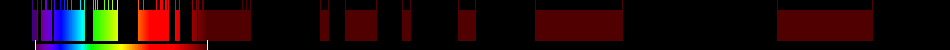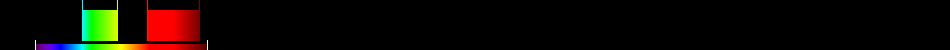# Information for Sensor and Index

## Formula

General Formula $\frac{{\mathrm{NDVI}}+0,5}{|{\mathrm{NDVI}}+0,5|}·\sqrt{|\left({\mathrm{NDVI}}\right)+0,5|}$ $\frac{\left(\frac{{\mathrm{14}}-{\mathrm{21}}}{{\mathrm{14}}+{\mathrm{21}}}\right)+0,5}{|\left(\frac{{\mathrm{14}}-{\mathrm{21}}}{{\mathrm{14}}+{\mathrm{21}}}\right)+0,5|}·\sqrt{|\left(\left(\frac{{\mathrm{14}}-{\mathrm{21}}}{{\mathrm{14}}+{\mathrm{21}}}\right)\right)+0,5|}$ Automatic

## Sensor

Name GLI Global Imager 36 375-12500 250-1000 98.6 Advanced Earth Observing Satellite 2 (ADEOS II) NASDA, NASA and CNES 2002-12-14 The mission ended in October 2003 (2003-10-23) after the satellite's solar panels failed. yes

## Index

Name Corrected Transformed Vegetation Index CTVI $\frac{{\mathrm{NDVI}}+0,5}{|{\mathrm{NDVI}}+0,5|}·\sqrt{|\left({\mathrm{NDVI}}\right)+0,5|}$ 490:570,640:760 Derived from specific sensor formula

## References